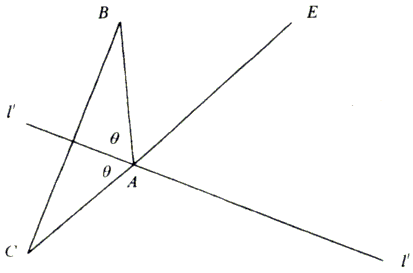# An Interesting Example of Angle Trisection by Paperfolding

### Sidney H. Kung January 6, 2011

Hull has shown a method (by H. Abe) of trisecting an angle via paperfolding (origami, in Japanese). Here we present a different one that was communicated to me years ago by Dr. Swend T. Gormsen, a retired Prof. of V.P.I. at Blacksburg, VA. I was able to prove the result only recently.

Let 2θ be the vertex angle of an isoceles triangle ABC (Figure 1) and let ll' be the perpendicular bisector of BC. AE is the extension of side CA.

Figure 1Fold the paper up (by Angle Trisection by Paper Folding, O6) so that points B and C fall upon AE (at the point B') and ll' (at the point C'), respectively, and then unfold. Denote by O the point of intersection of ll' and the line of crease zz' (Figure 2). Construct line segment BO. Then we have ∠AOB = 2θ/3.

Figure 2### Proof

By symmetry we see that OB = OB' = OC = OC'. So, B, B', C, and C' lie on a circle centered at O. Thus, arcBC = arcB'C'. Construct C'E' parallel to CE intersecting the circle at N. Let M be the antipode of C'.

Then we have arcCN = arcB'C'. Further,

 θ = ∠MC'N = (arcMC + arcCN)/2 = (arcBC/2 + arcB'C')/2 = 3·arcBC/4.

Therefore, ∠AOB = arcBC/2 = 2θ/3.

### References

1. T. Hull, Project Origami: Activities For Exploring Mathematics, A.K. Peters Ltd. (2007) 49-50.Paper Folding Geometry